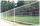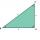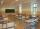# Christmas napkins

The girls embroidered Christmas napkins. Each napkin had the shape of a triangle with sides of 5 dm, 60 cm, and 800 mm. How many cms did the girls sew if they made 15 napkins?

x =  2850 cm

### Step-by-step explanation:

Try calculation via our triangle calculator.Did you find an error or inaccuracy? Feel free to write us. Thank you!Tips to related online calculators
Do you want to convert length units?

#### You need to know the following knowledge to solve this word math problem:

We encourage you to watch this tutorial video on this math problem:

## Related math problems and questions:

• SquaresCalculate the area of square and result round to square decimeters. a) a = 1,52 dm b) a = 13 268mm c) a = 562 cm d) a = 1,52 m
• The bookThe book has 280 pages. Each side is a rectangle with sides 15 cm and 22 cm. a) how many leaves has the book? b) at least how many square meters of paper should be used to production this book?
• Two sides paintThe door has the shape of a rectangle with dimensions of 260cm and 170cm. How many cans of paint will be needed to paint this door if one can of paint cover 2m2 of the area? We paint the doors on both sides.
• RectanglesHow many different rectangles with sides integers (in mm) have a circumference exactly 1000 cm? (a rectangle with sides of 50cm and 450cm is considered to be the same as a rectangle with sides of 450cm and 50cm)
• Children poolThe bottom of the children's pool is a regular hexagon with a = 60 cm side. The distance of opposing sides is 104 cm, the height of the pool is 45 cm. A) How many liters of water can fit into the pool? B) The pool is made of a double layer of plastic film
• WindowsCalculate the masonry area to build a wall with dimensions of 9 m × 4 m with 4 windows of size 64 cm × 64 cm.
• TableclothsThe restaurant has sixty-two square tablecloths with a side length of 150 cm and 36 rectangular tablecloths with dimensions of 140 cm and 160 cm. A) How many meters of hemming ribbon will be needed if we add 50 cm to each tablecloth? B) The ribbon sale in
• PexesoDrawing paper has dimensions of 60cm and 840mm. Pupils have to divide it into squares so that they can make a pexeso. What dimension must squares have if their side is to be larger than 3cm and less than 10cm?
• Do you solve this?Determine area S of rectangle and length of its sides if its perimeter is 102 cm.
• The roomThe room has a cuboid shape with dimensions: length 50m and width 60dm and height 300cm. Calculate how much this room will cost paint (a floor is not painted) if the window and door area is 15% of the total area and 1m2 cost 15 euro.
• Four prismsQuestion No. 1: The prism has the dimensions a = 2.5 cm, b = 100 mm, c = 12 cm. What is its volume? a) 3000 cm2 b) 300 cm2 c) 3000 cm3 d) 300 cm3 Question No.2: The prism base is a rhombus with a side length of 30 cm and a height of 27 cm. The height of t
• MidpointsTriangle ABC with sides a = 5 cm, b = 3 cm, c = 40mm has a midpoint of K, L, M. How many centimeters is long perimeter of parallelogram KBLM?
• TailorTailor has an estimated 11 meters of 80 cm wide fabric. He will shape wholly 7 rectangles measuring 80 x 150 cm and nothing fabric does not remain. How many fabric he have?
• Fence of the gardenThe garden has dimensions of 5m and 400cm. How many meters meshes are needed for fencing the plot?
• Parallelogram perimeterThe ABC triangle with sides a = 5cm, b = 3cm, c = 40mm has the center of the sides of the K,L,M. How many cm have the KBLM parallelogram perimeter?
• SpeedsGeorge pass on the way to school distance 200 meters in 165 seconds. What is the average walking speed in m/s and km/h?
• Triangle ABCIn a triangle ABC side b measure 10 cm less than the side a and side b is half of the side c. Calculate the length of sides if the circumference of the triangle is 42 cm.